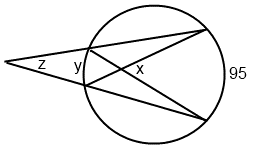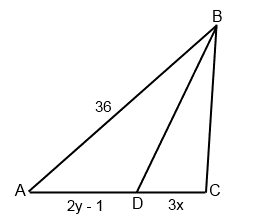+91-22-62437100 / 01 | help@reachivy.com

# GMAT Data Sufficiency Quiz 1

Don’t let the Data Sufficiency bog you down. If you are not sure how prepared you are, take a sample Data Sufficiency test now and determine whether you are ready to take the plunge.

Instructions: This data sufficiency problem consists of a question and two statements, labeled (1) and (2), in which certain data are given. You have to decide whether the data given in the statements are sufficient for answering the question. Using the data given in the statements, plus your knowledge of mathematics and everyday facts (such as the number of days in July or the meaning of the word counterclockwise), you must indicate whether:

Statement (1) ALONE is sufficient, but statement (2) alone is not sufficient to answer the question asked.
Statement (2) ALONE is sufficient, but statement (1) alone is not sufficient to answer the question asked.
BOTH statements (1) and (2) TOGETHER are sufficient to answer the question asked, but NEITHER statement ALONE is sufficient to answer the question asked.
Statements (1) and (2) TOGETHER are NOT sufficient to answer the question asked, and additional data specific to the problem are needed.

To begin click Next

Question 1:

If Circle B is larger than Circle A and Circle A is inscribed in Square A. Is the ratio of the area of Circle B to the area of Circle A less than two?
(1) The distance between Circle A and Circle B is 1.
(2) Square A is inscribed in Circle B.
Question 2:

If each term in an infinite sequence is found by the equation Sx = 8x – 6, and S1 = 2, is every term in the sequence divisible by y?
(1) y is an even integer.
(2) At least 2 terms in the sequence are divisible by y.

Question 3:

Is the absolute value of x equal to z less than y?
(1) The sum of x and y is equal to z.
(2) x is a negative number.

Question 4:

What is the value of (a - c)2?
(1) ac = 1
(2) (a + c)2 = 26

Question 5:

Three rectangular tiles are fitted together to form a larger rectangle. What is the ratio of the width to the length of the larger rectangle?
(1) The area of the larger rectangle is 36.
(2) Each of the three rectangular tiles have the same dimensions.

Question 6:

Triangle ABC lies on a coordinate grid such that the y-value of point A is greater than the y-value of point C, and C lies on the origin. If point B lies somewhere in Quadrant I but not on either axis and AB > BC, is the area of the triangle < 30?
(1) The coordinates of point B are (5, 6).
(2) The coordinates of point A are (0, 9).

Question 7:

If L = 4/(5b), M = 3/a, N = 2/b, and the product of a and b is not equal to zero, what is the value of X is X is equal to the value of M divided by the sum of L and N?
(1) b = 4a
(2) a = 2/3

Question 8:

Is 2 raised to the power of integer x is more than the product of x and its absolute value?
(1) x is a negative number
(2) x = –27

Question 9:What is the value of minor arc y?
(1) Angle x = 70
(2)Angle z = 25
Question 10:What is the value of y > 28?
(1) BC = 2/3(AB)
(2) Angle ABD = Angle DBC

You have answered all the questions in the quiz. Press Submit to view your score